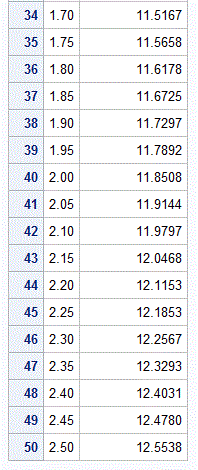## Cosmologically Distant Objects Appear Magnified

George F. R. Ellis writes in section 2.3.3 of his outstanding survey paper, Issues in the Philosophy of Cosmology:

…there is a minimum apparent size for objects of ﬁxed physical size at some redshift zc = z depending on the density parameter and the cosmological constant.  The past light cone of the observer attains a maximum area at z; the entire universe acts as a gravitational lens for further oﬀ objects, magnifying their apparent size so that very distant objects can appear to have the same angular size as nearby ones.  For the Einstein-de Sitter universe, the minimum angular diameter is at z = 1.25; in low density universes, it occurs at higher redshifts.

Electromagnetic radiation such as visible light that we observe from a source that is in motion relative to us (the observer) experiences a change in wavelength that is given byThis is called redshift and is positive for a source that is moving away from us and negative for a source that is moving towards us.  The higher the relative speed toward or away from us, the greater the magnitude of the redshift.  Superimposed upon the kinematic velocities of individual galaxies relative to our Milky Way galaxy, since 1929 we have known that there is a cosmological redshift (called the Hubble flow) that is always positive and increasing in magnitude with increasing distance between any two galaxies.  In the nearby universe, the redshift (or blueshift) from kinematic velocities (often referred to as “peculiar velocities”) swamp the contribution from the Hubble flow, so some galaxies are actually approaching each other.  A good example of this is M31 and the Milky Way galaxy.  For more distant galaxies, however, the cosmological redshift swamps any contribution from the kinematic velocities.  Thus, redshift becomes a useful proxy for distance at cosmological distances.

From our everyday experience, we know that the further away an object is, the smaller is its angular size.  However, there comes a point where the angular size of an object reaches a minimum, and at even greater distances, its angular size increases!  As George Ellis states above, the entire universe acts as a gravitational lens to magnify distant objects.

Michael Richmond presents an equation for angular size as a function of redshift (based on some classical assumptions about the structure of the universe).  In his equation, the angular size of an object also depends upon the value we choose for H0, the Hubble constant, the matter density parameter, ΩM, and, of course, the physical size of the object of interest.Let’s work through an example using this equation.  The visible part of the Andromeda Galaxy is estimated to be about 220,000 light years across.  In megaparsecs, that is 0.0675.  This is the value we will use for S.

For the Hubble constant, H0, let use a recent result: 71.9 km/s/Mpc.

And, for the matter density parameter, ΩM, let’s use 1.0.  This indicates that we live in a universe that has just enough matter for the universe to eventually recollapse, were it not for dark energy.  Though Richmond’s equation above only applies to a matter-dominated universe where the dark energy density parameter ΩΛ is zero, as George Ellis indicates above, a minimum angular diameter is still reached in a universe with dark-energy (i.e. low density universe), only this occurs at a higher redshift than that presented here.

I have not been able to find or derive a more general equation for angular size as a function of redshift that will work for a dark-energy-dominated universe (perhaps a knowledgeable reader will post a comment here providing some insight into this issue), but it will be a useful exercise to continue with the calculation assuming the matter-dominated Einstein-de Sitter universe.

Casting Michael Richmond’s equation into the following SAS program, I was able to find that the Andromeda galaxy would reach a minimum angular size of 11.3 arcseconds at z = 1.25, as shown below.In principle, measuring the angular size of a “standard” object at various redshifts could allow us to determine what kind of universe we live in.  But there’s a problem.  As we look further out into space we are also looking further back in time, so there is no guarantee that a “standard” object in today’s universe (say, a spiral galaxy such as M31) would have looked the same or even existed billions of years ago.

References
Ellis, G. F. R. 2006, Issues in the Philosophy of Cosmology, Philosophy of Physics (Handbook of the Philosophy of Science), Ed. J. Butterfield and J. Earman (Elsevier, 2006), 1183-1285.
[http://arxiv.org/abs/astro-ph/0602280]

Richmond, Michael, Two classic cosmological tests
[http://spiff.rit.edu/classes/phys443/lectures/classic/classic.html]